# Acta Universitatis Danubius. Œconomica, Vol 10, No 5 (2014)

A Type of a Rational Production Function

Catalin Angelo Ioan1, Gina Ioan2

Abstract: The article deals with a particular rational production function of two factors with constant scale return. It were determined from the compatibility conditions with the axioms of production function all the cases for a such function.

Keywords: production function; marginal productivity; average productivity

JEL Classification: D00

1. Introduction

In what follows we shall presume there is a certain number of resources, supposedly indivisible needed for the proper functioning of the production process.

We define on R2the production space for two resources: K – capital and L - labor as SP=(K,L)K,L0 where xSP, x=(K,L) is an ordered set of resources and we restrict the production area to a subset DpSP called domain of production.

It is called production function an application Q:DpR+, (K,L)Q(K,L)R+ (K,L)Dp.

For an efficient and complex mathematical analysis of a production function, we impose a number of axioms both its definition and its scope.

1. The domain of production is convex;

2. Q(0,0)=0 (if it is defined on (0,0));

3. The production function is of class C2 on Dp that is it admits partial derivatives of order 2 and they are continuous;

4. The production function is monotonically increasing in each variable;

5. The production function is quasiconcave that is: Q(x+(1-)y)min(Q(x),Q(y)) [0,1] x,yRp.

In a preceding paper (), one of the authors define a rational production function with constant return to scale as:

Q:DpR2R+, (K,L)Q(K,L)R+ (K,L)Dp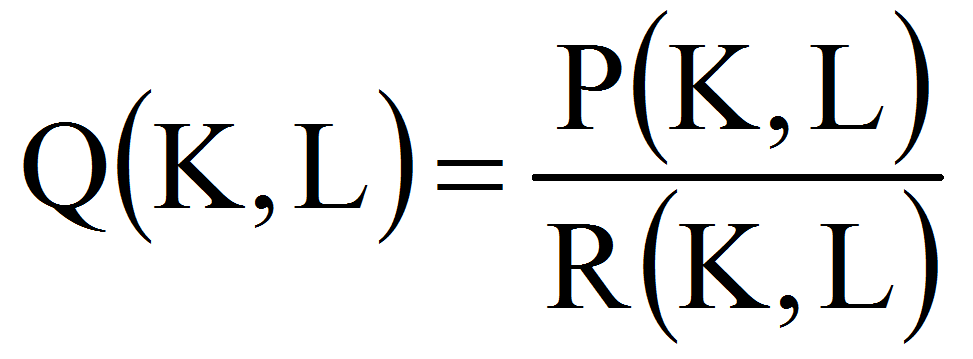K,L0

where P and R are homogenous polynomials in K and L, deg P=n, deg R=n-1, n2.

The compatibility conditions for that function to be of production were (from theorem 2):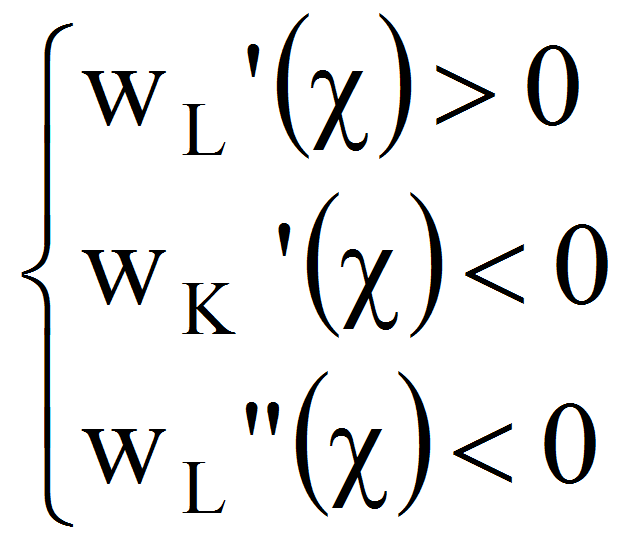where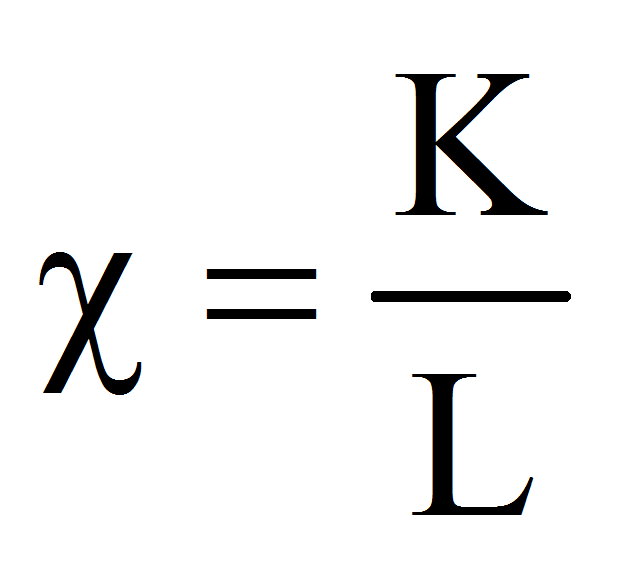and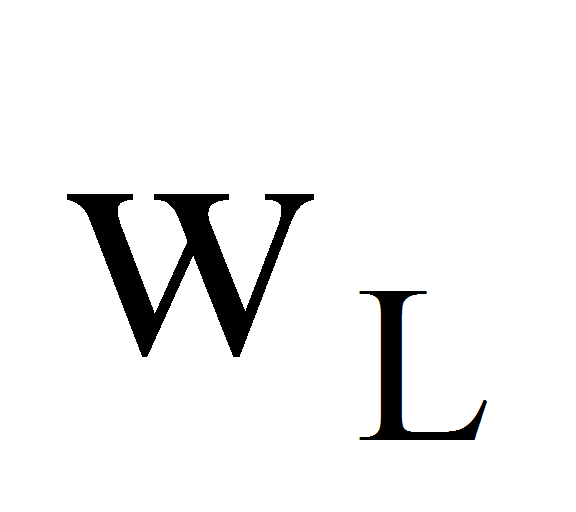,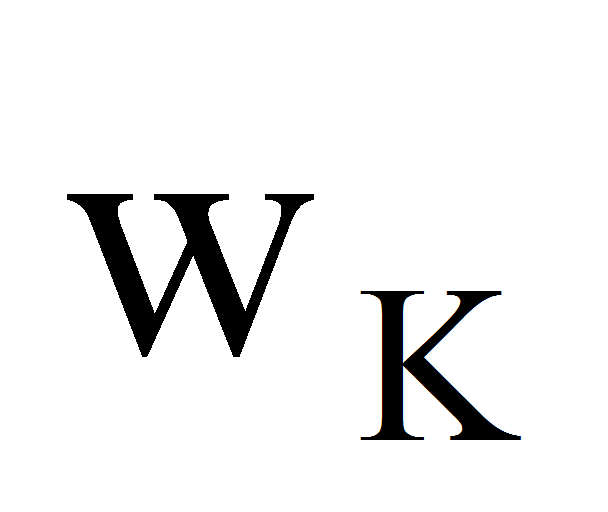are the average productivity relative to L and K respectively.

1. A Type of a Rational Production Function

Let now: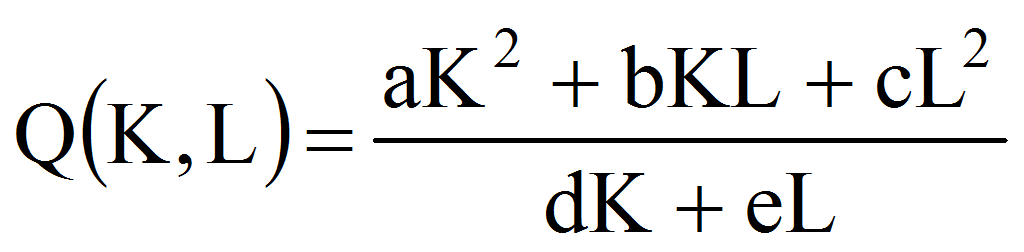, a,d0

We shall suppose that d=1 with loss of generality, after a simplification of the ratio with d.

Therefore, let: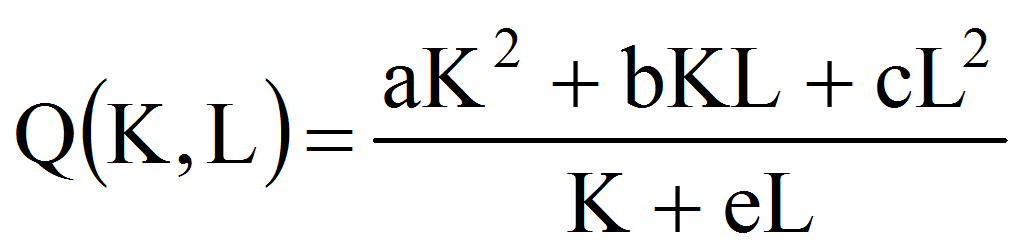=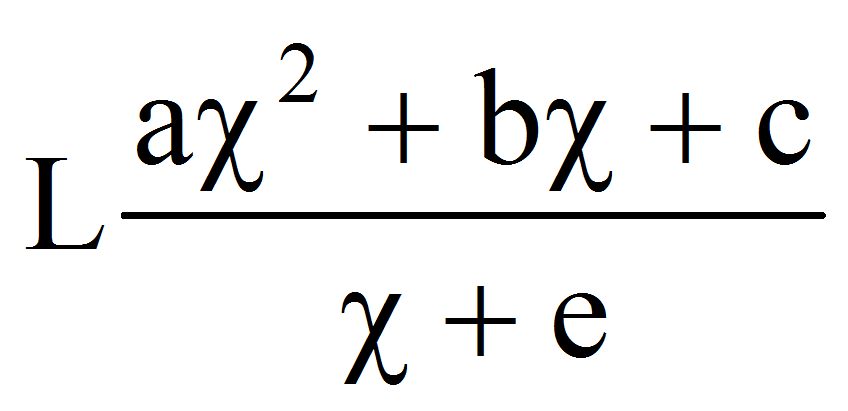.

The average productivity relative to K and L are: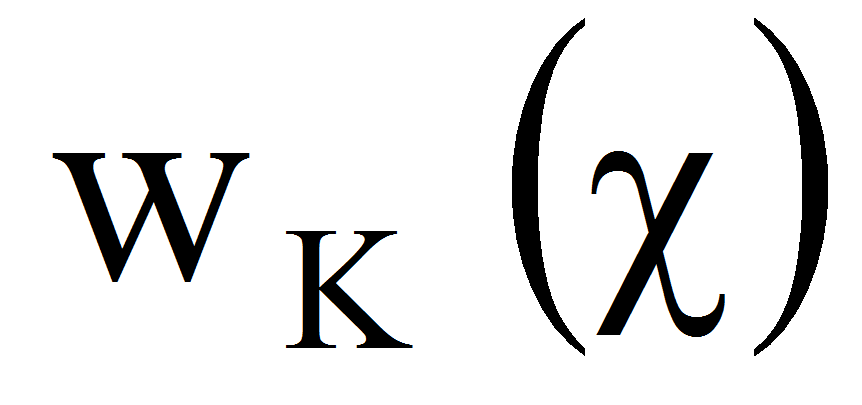=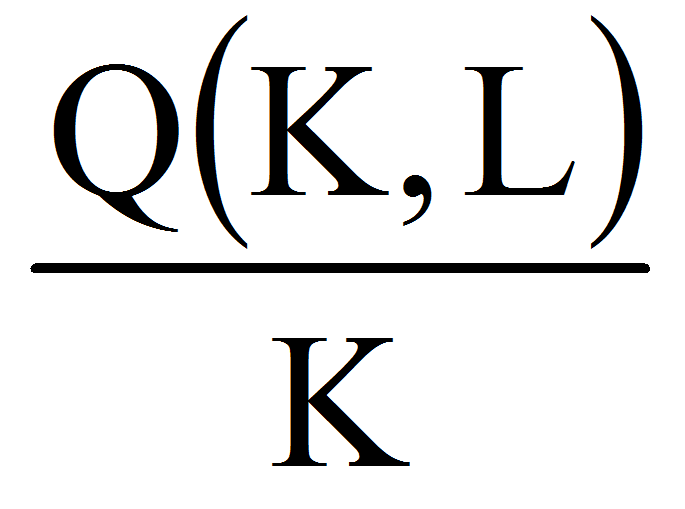=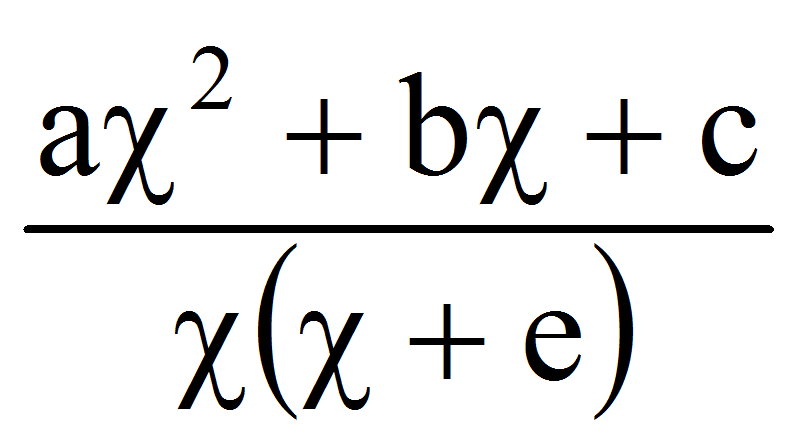,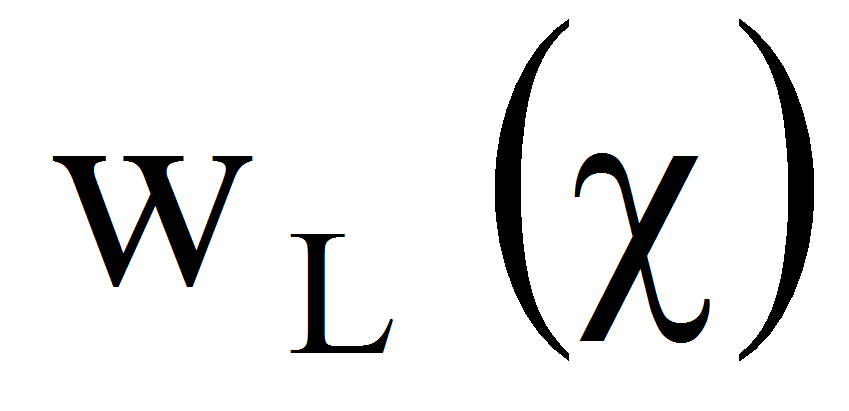=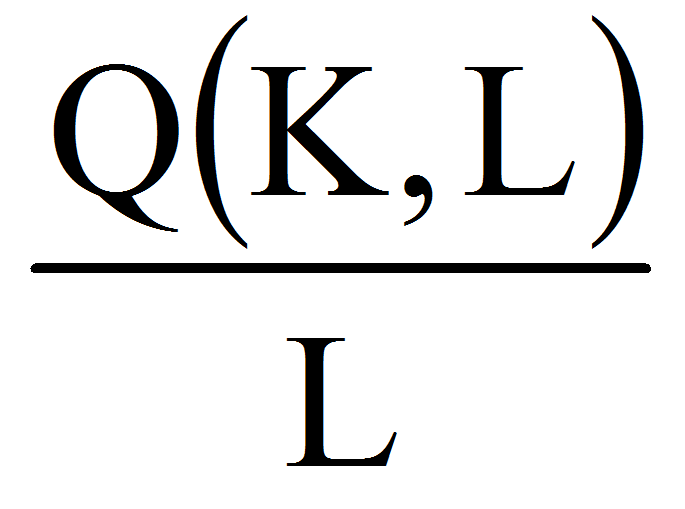=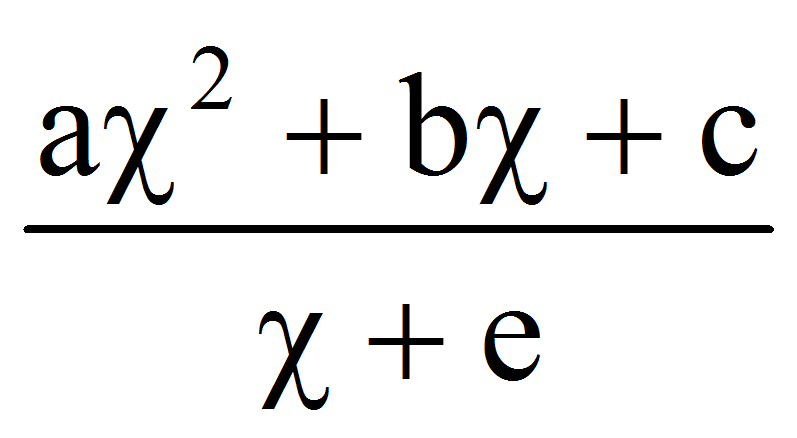The first and the second derivatives are: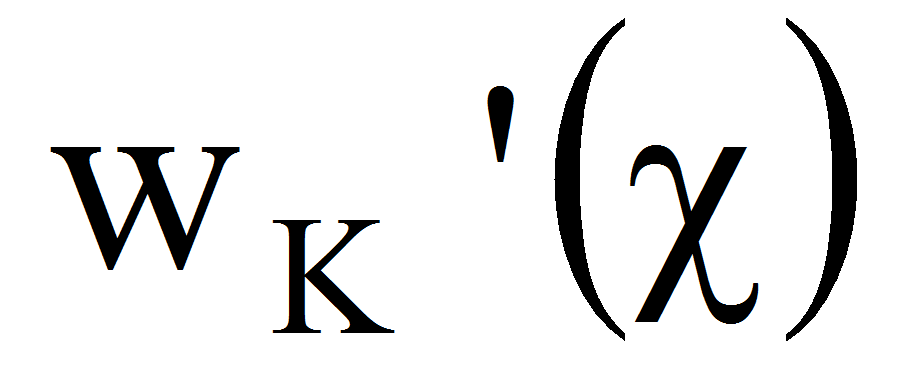=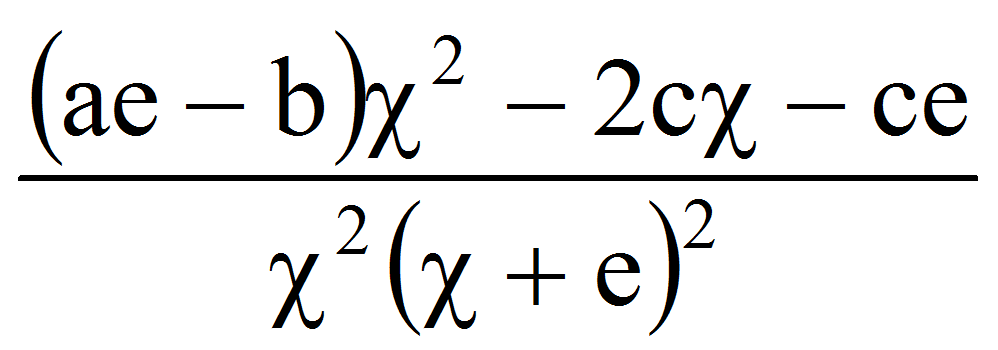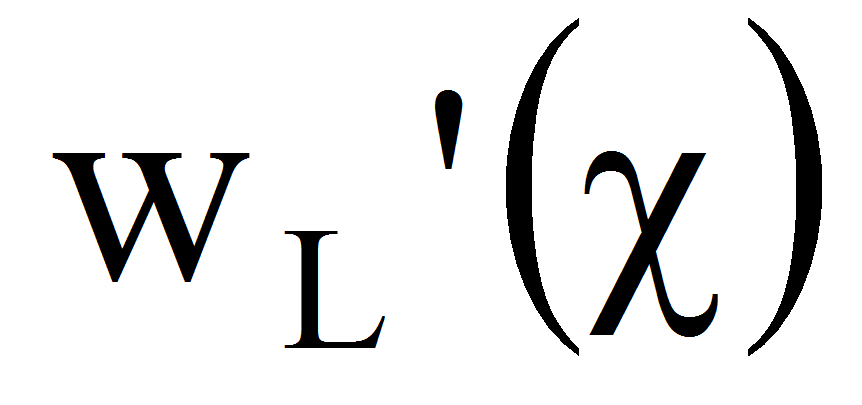=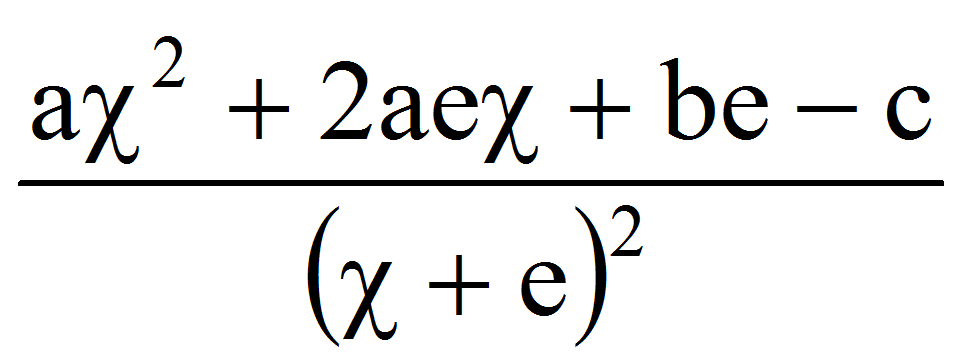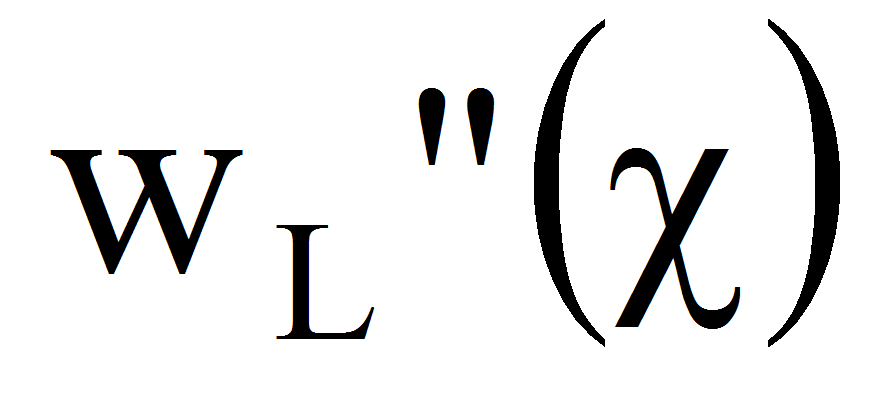=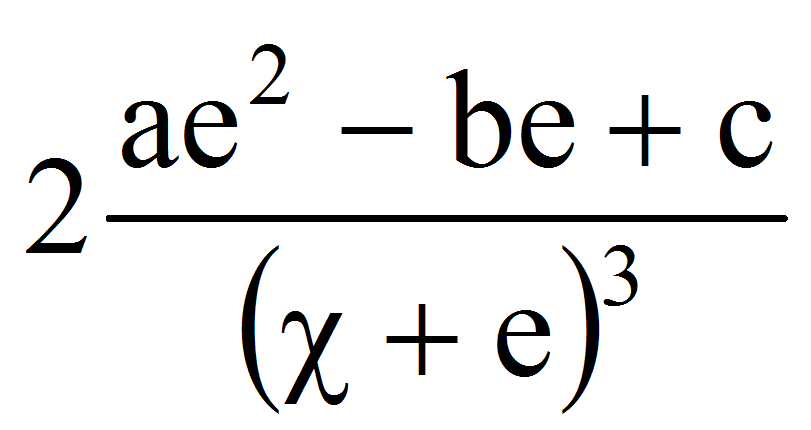The compatibility conditions become: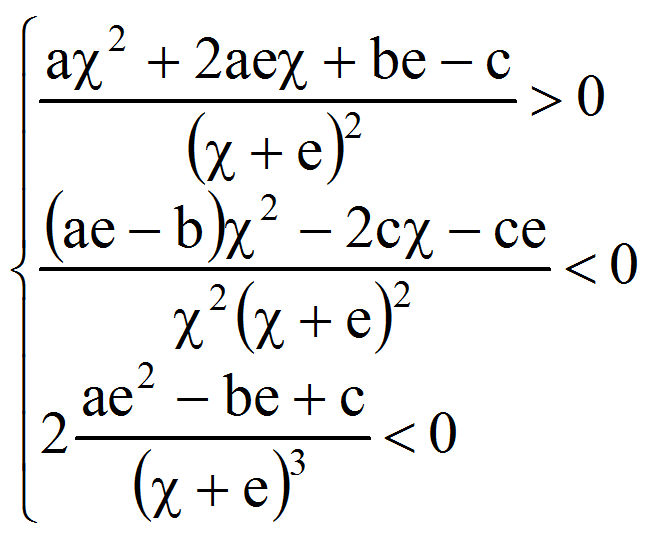or after simplifying: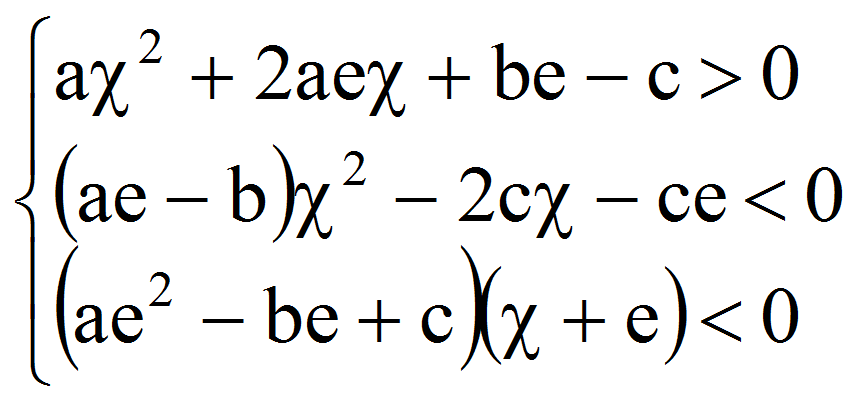Let now the transformation: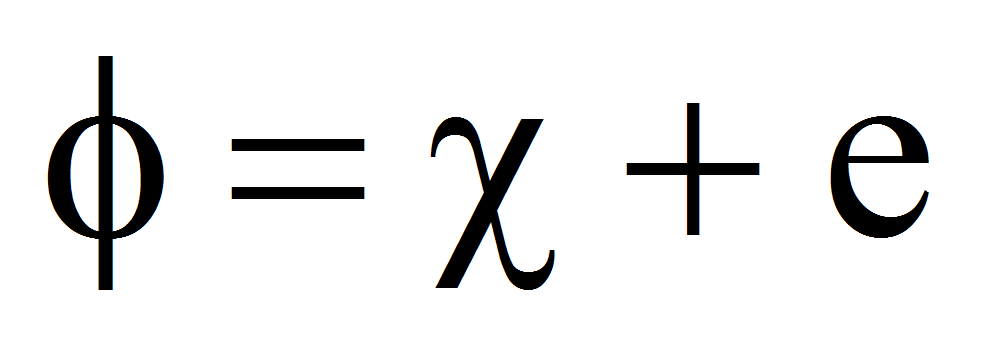, therefore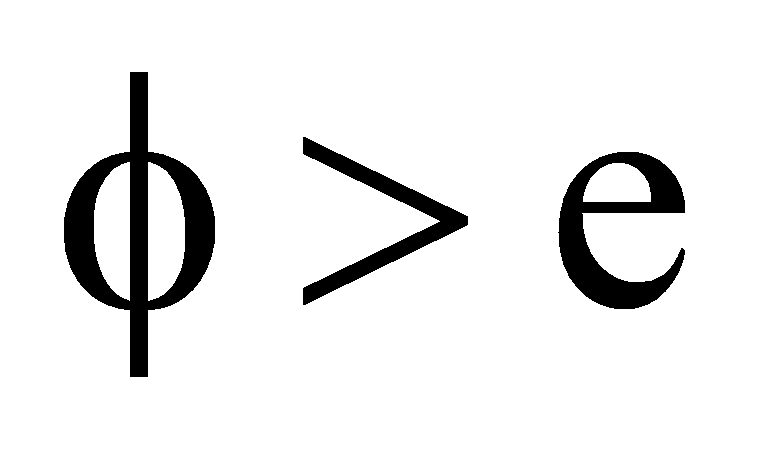and also: g=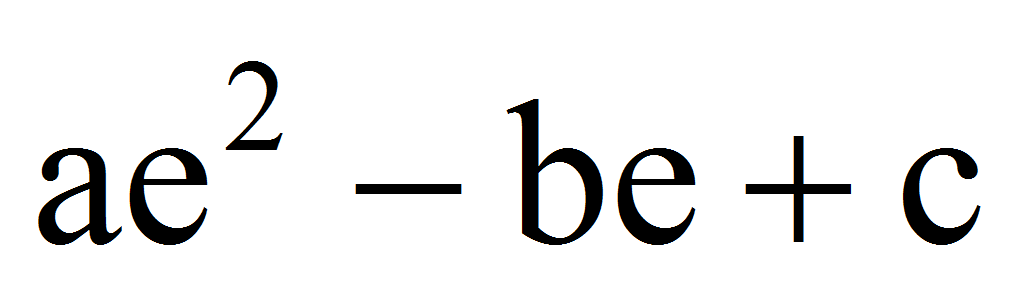. The conditions become: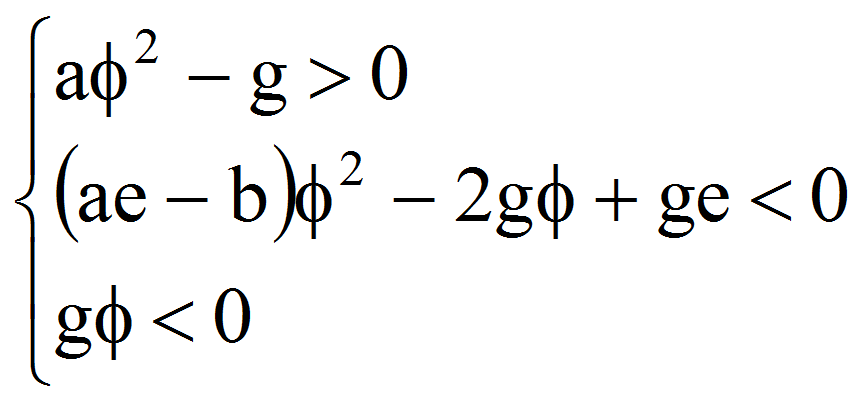Case 1: g0

From the third inequality, we have that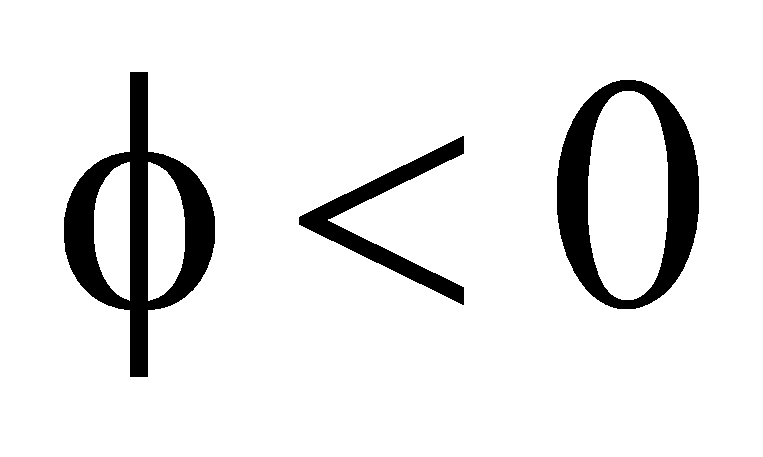. From the first: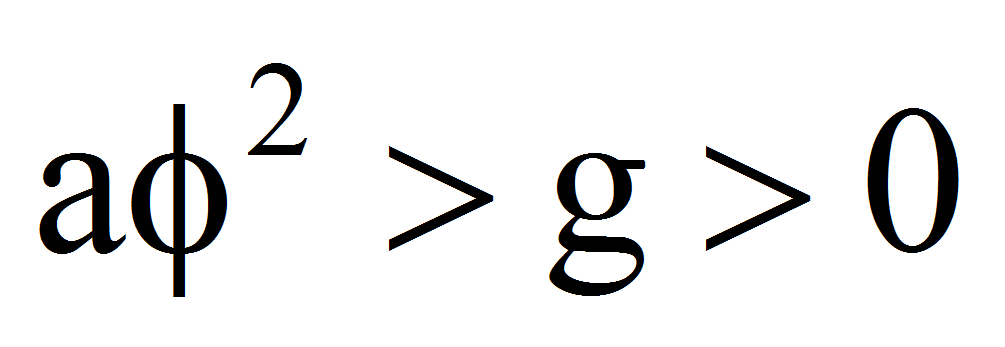therefore a0. Also: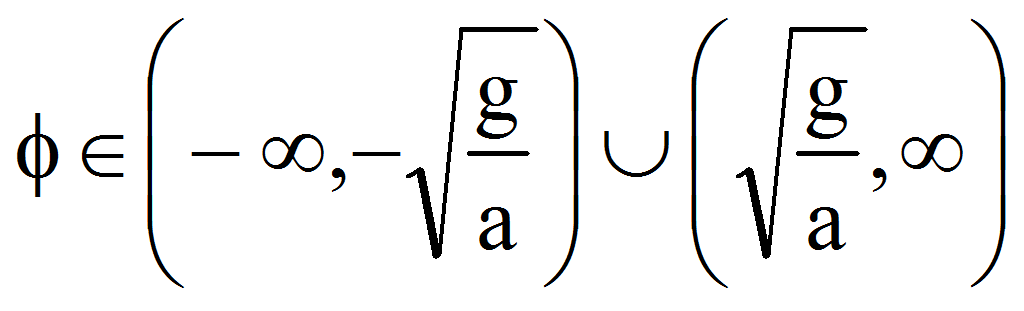and becausewe get: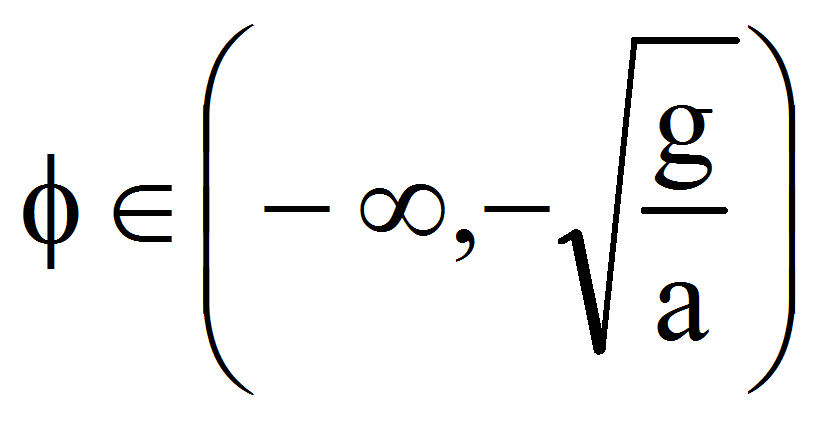or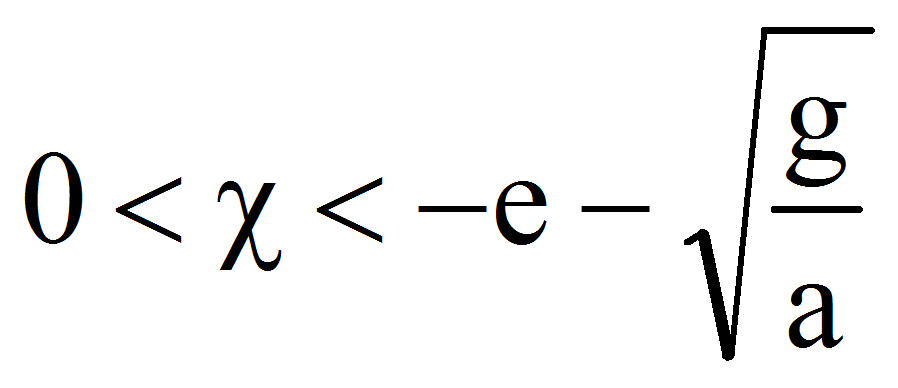.

But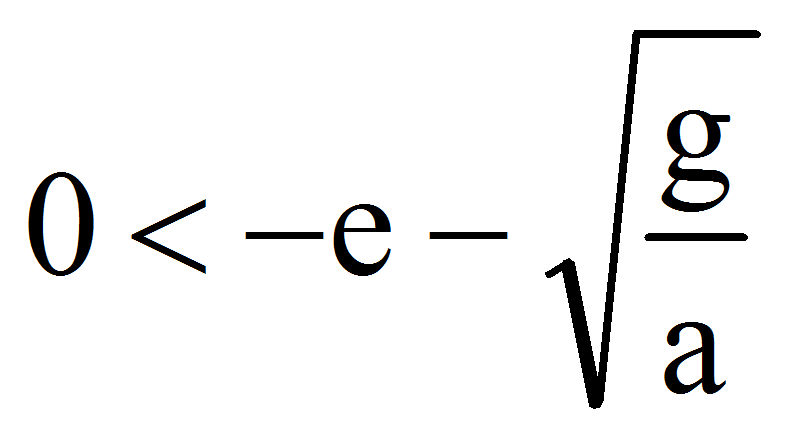is equivalent with: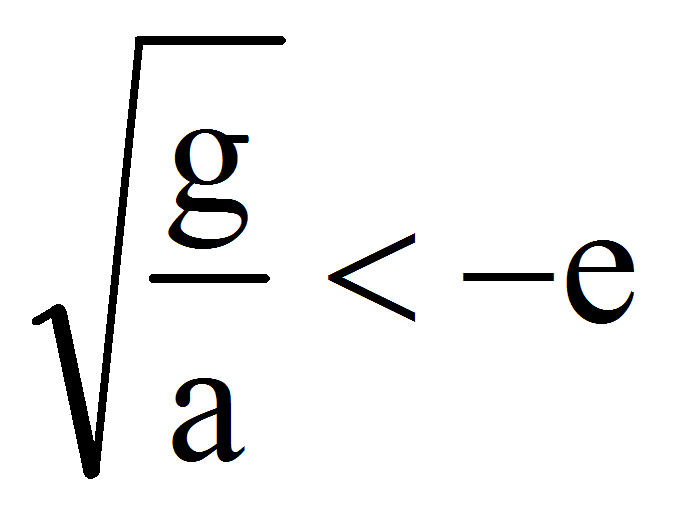therefore e0 and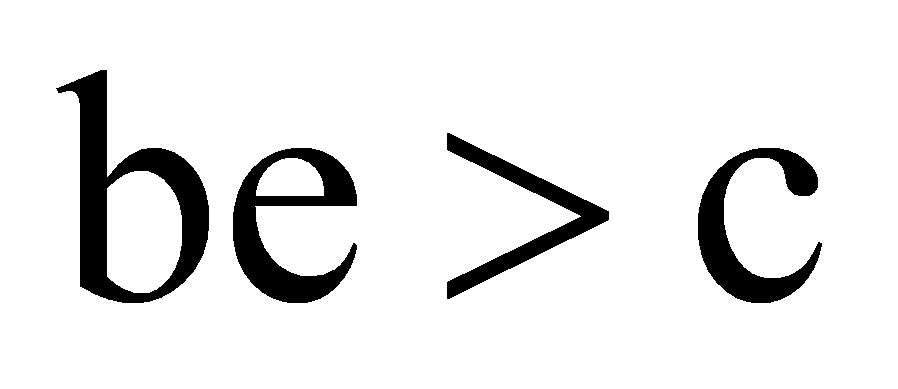.

Analysing the inequality: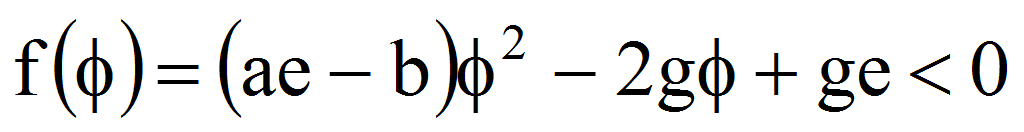we have first =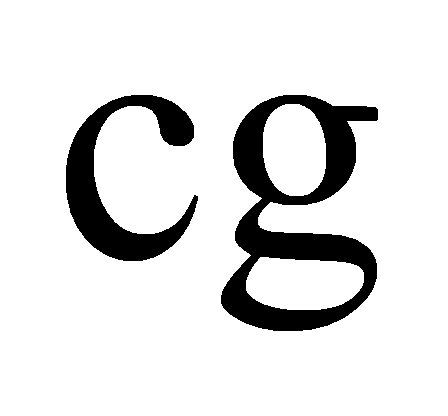.

If c0 then 0 therefore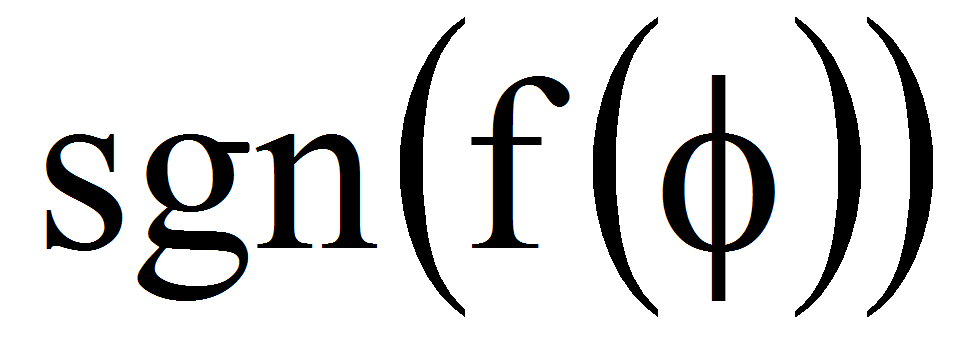=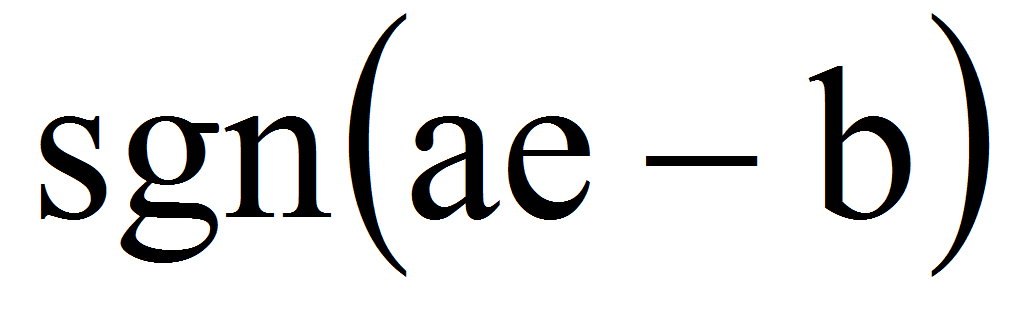=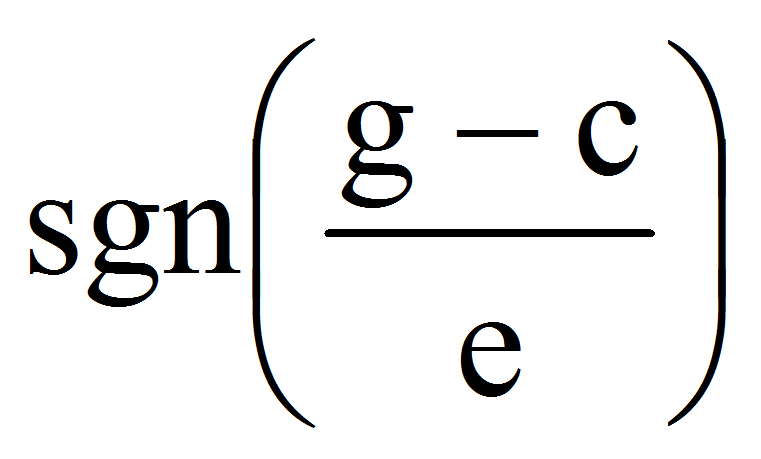=-1. In consequence: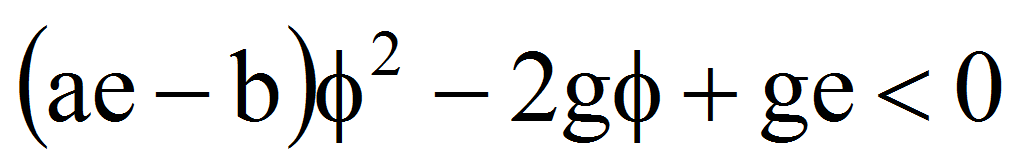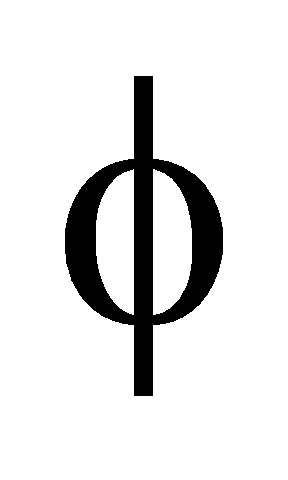R.

If c0 then 0. The roots of the equation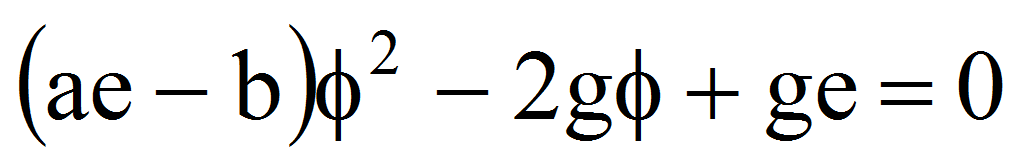are: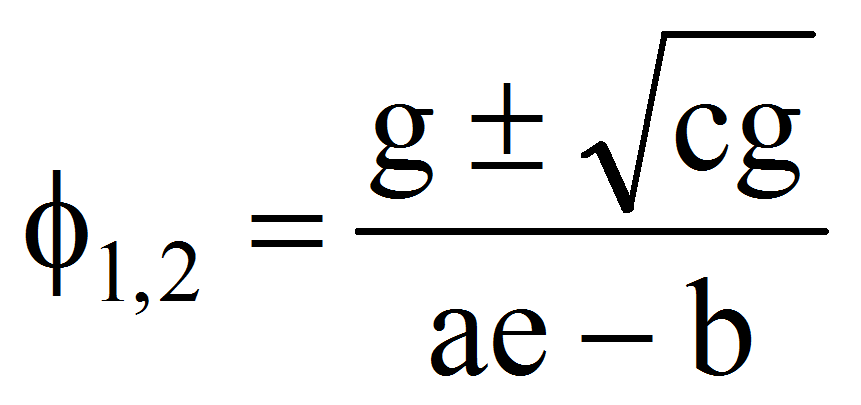therefore if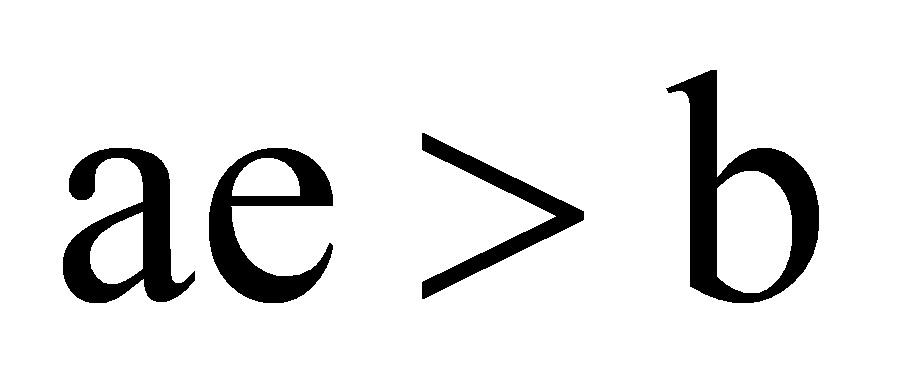then: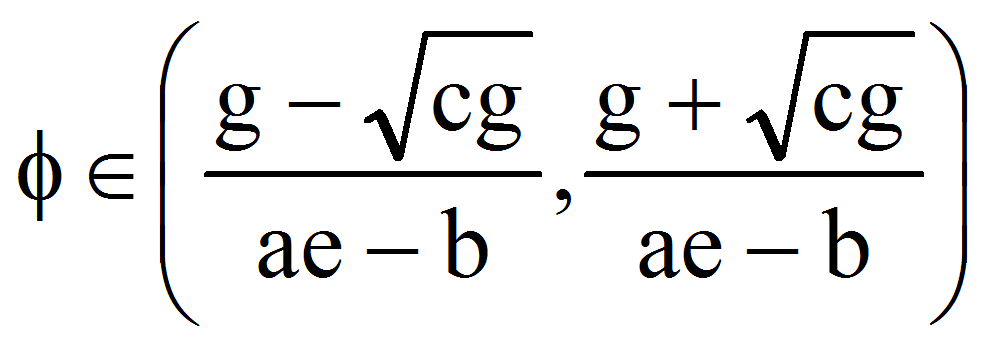which is possible becauseif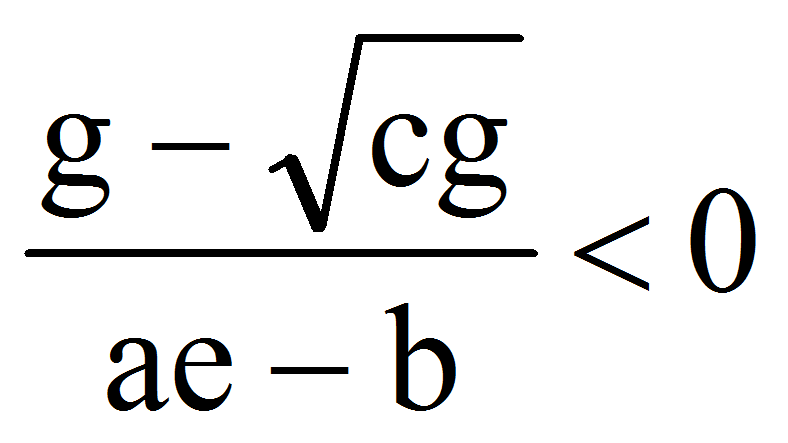that is: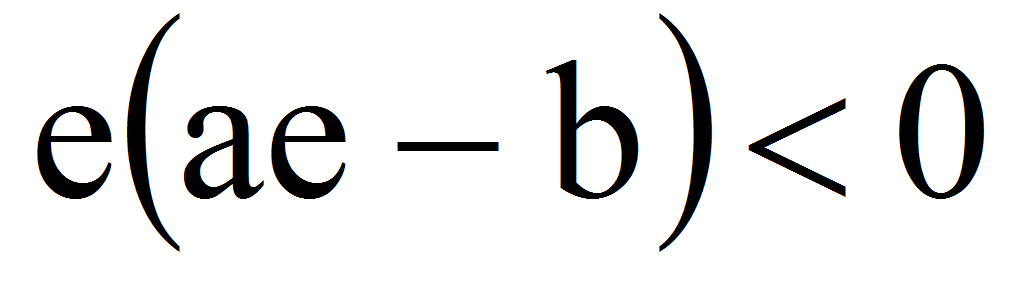which is true. In this case: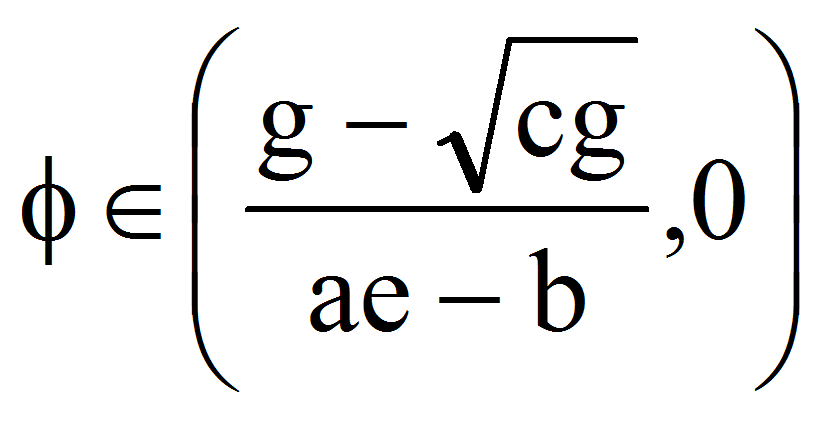or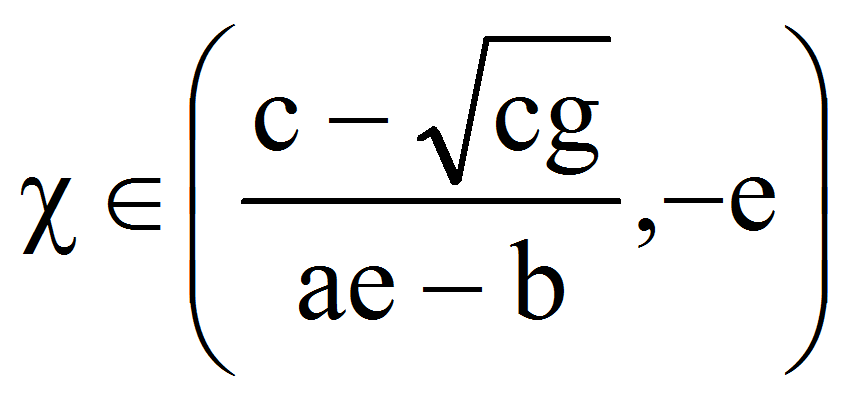.

If now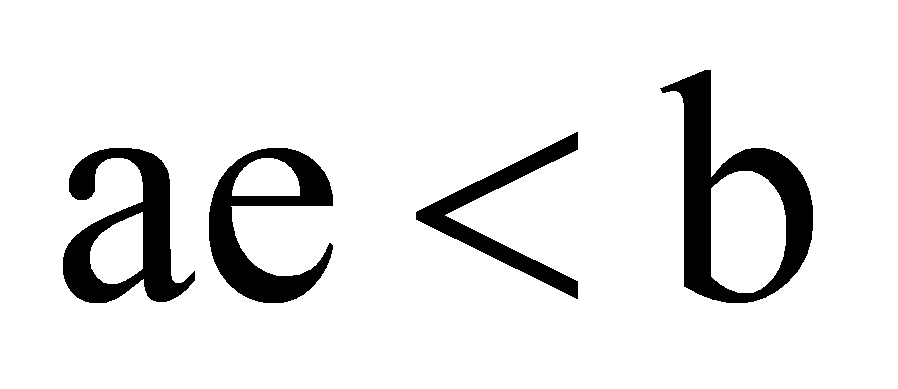then: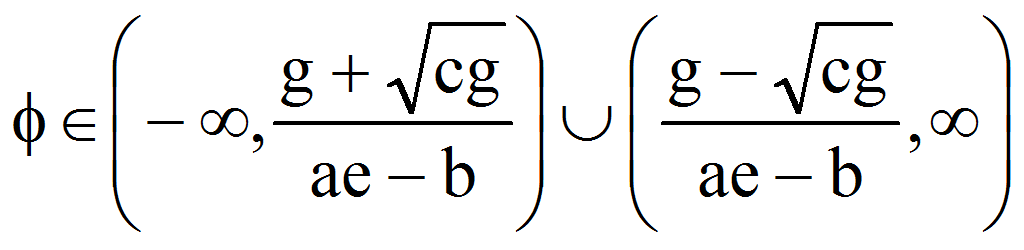. Because, in this case: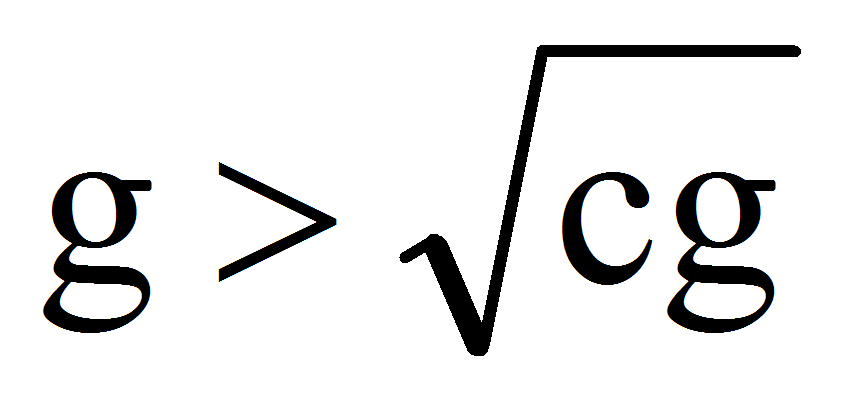we finally find that: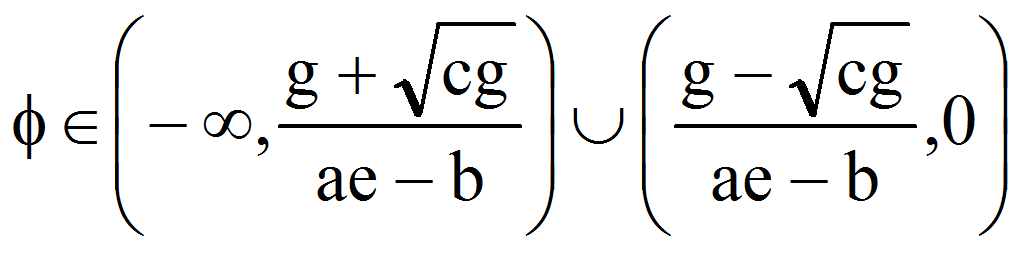or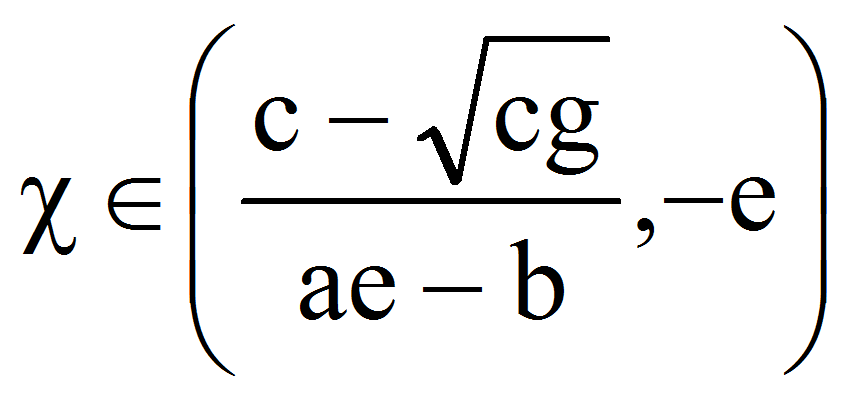.

If ae=b then: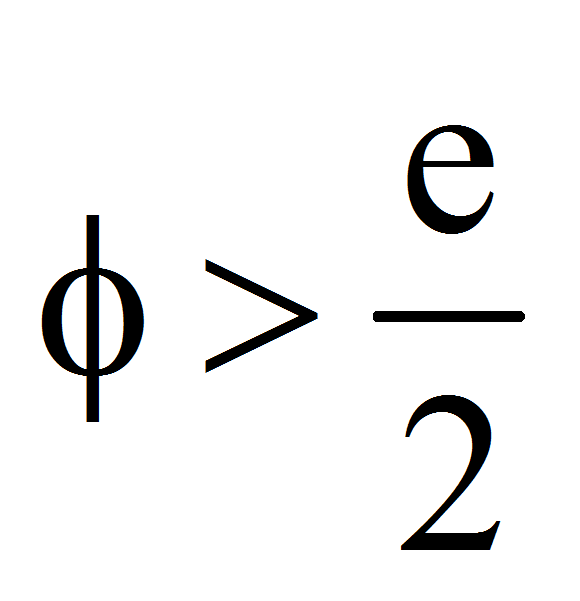which is true because e0. In this case:.

Case 2: g0

From the third inequality, we have that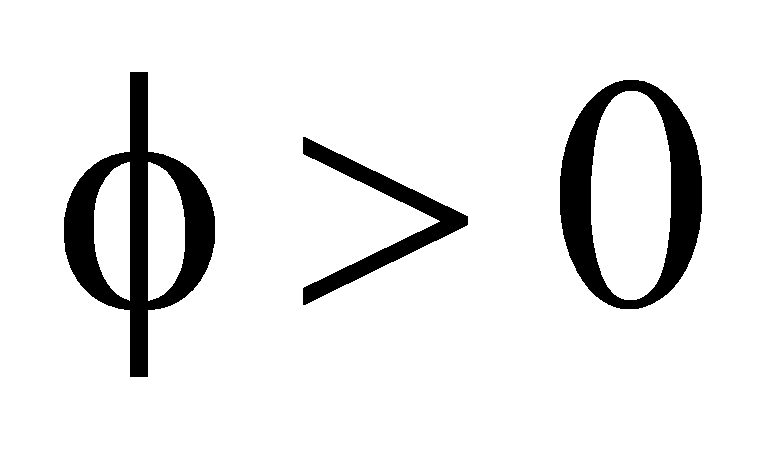. From the first: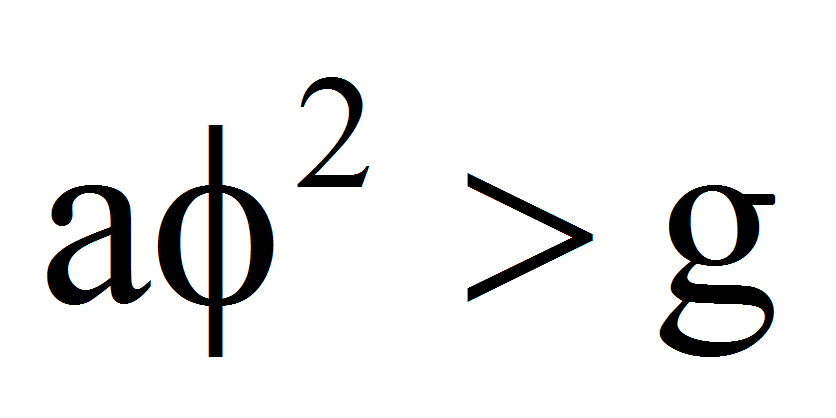.

If now a0 the inequality holds for allR. From the relation: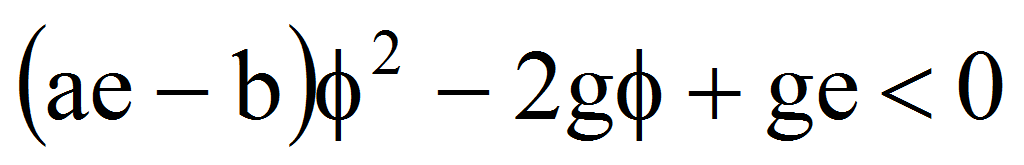we have =.

If c0 we obtain 0 therefore ifthen the inequality holds for allR. Ifthen:.

If ae=b then: g=c0 – contradiction.

If now c0 we have: 0 therefore, if:or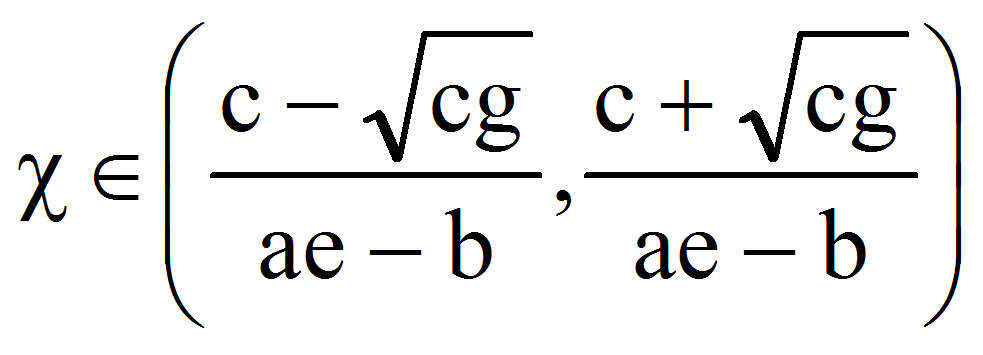If e0 then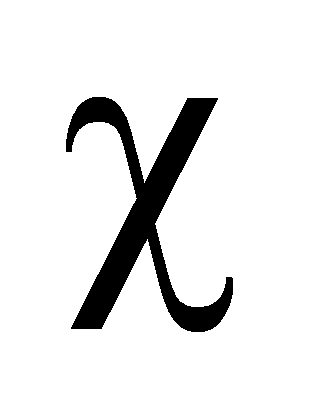0 therefore. If e0 then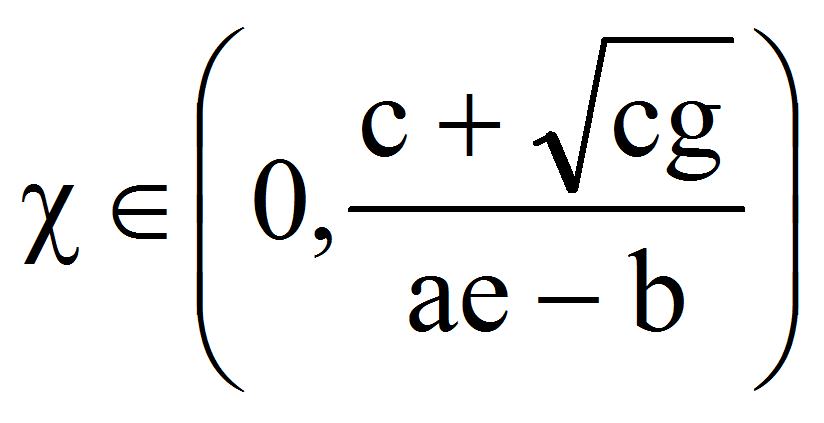.

If now:that is: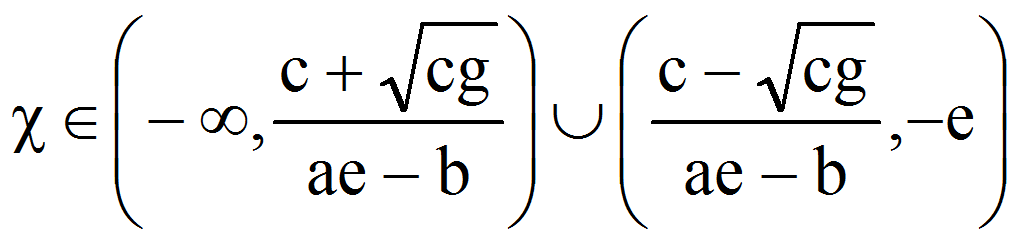.

If e0 then0 therefore. If e0 then: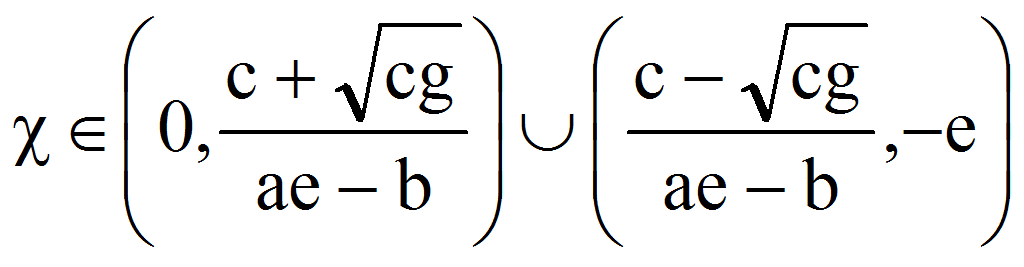.

If c=0 then =0 and we must haveand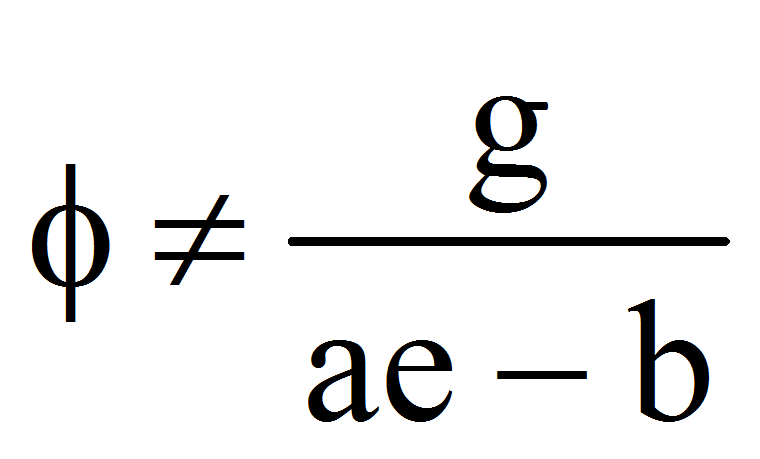or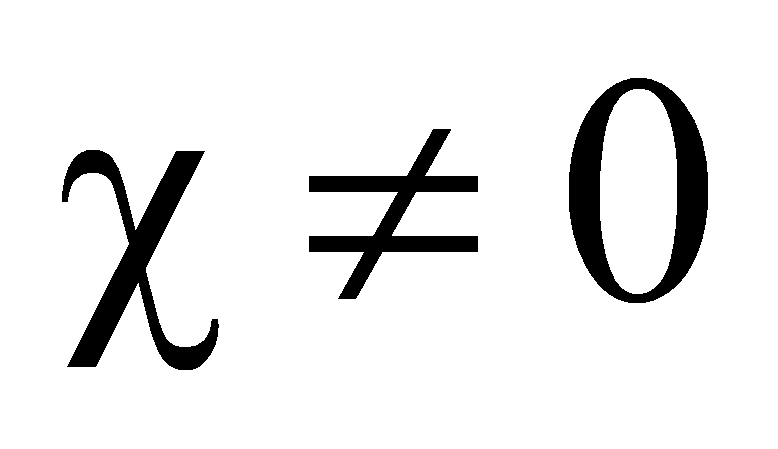.

Let suppose now that a0. In this case, from the first: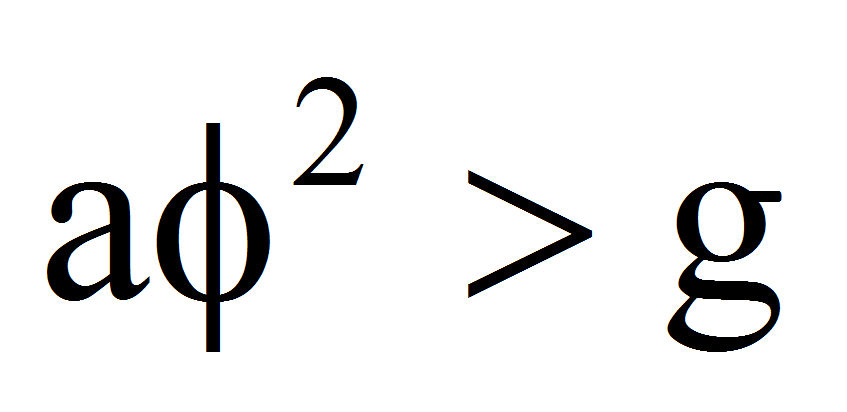therefore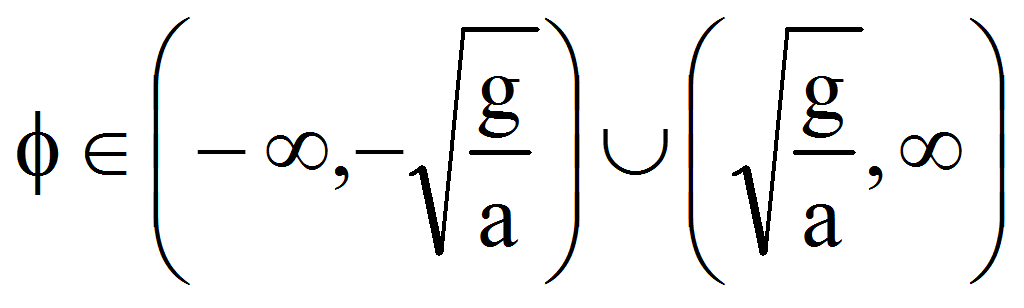and because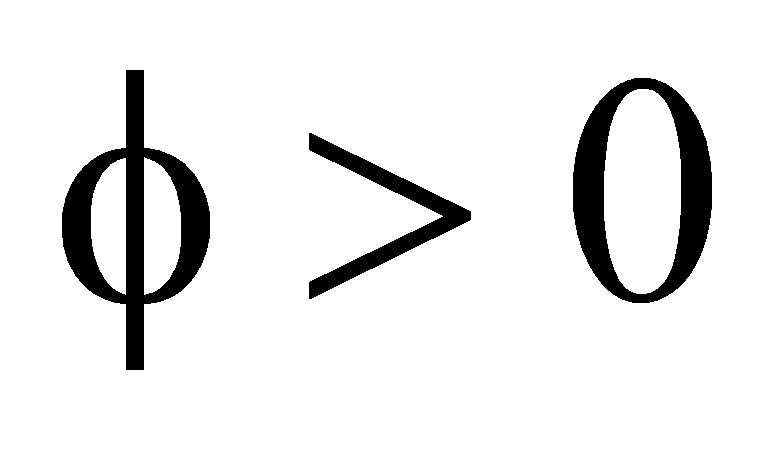we get: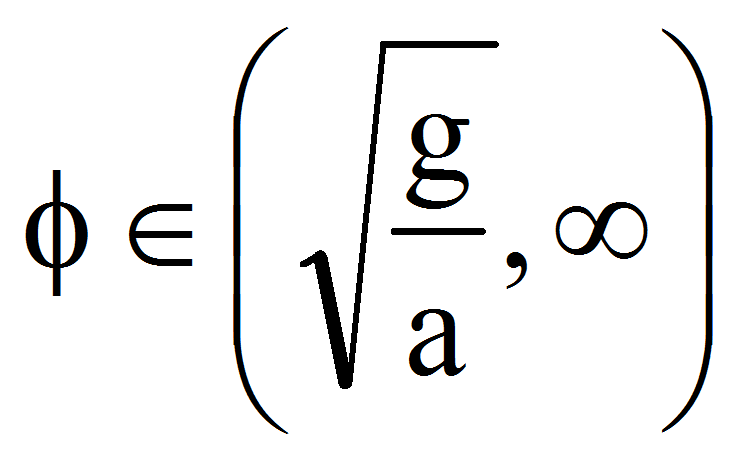or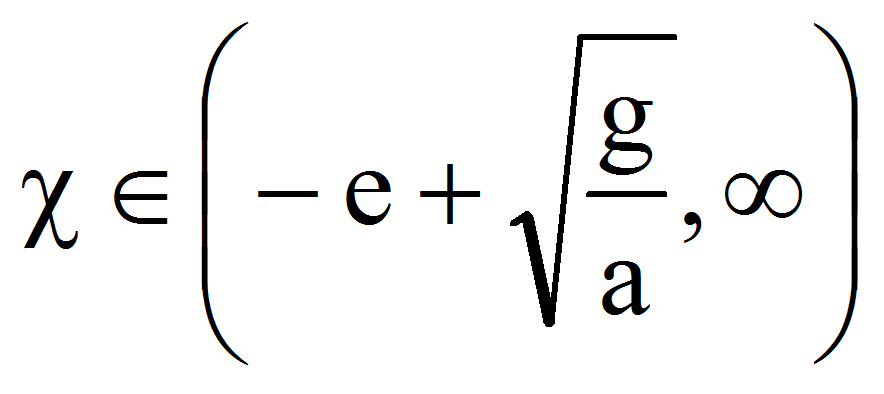.

For the inequalitywe have =.

If c0 we obtain 0 therefore ifthen the inequality holds for allR. Ifthen:.

If ae=b then: g=c0 – contradiction.

If now c0 we have: 0 therefore, if:or